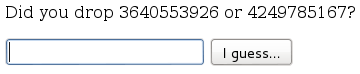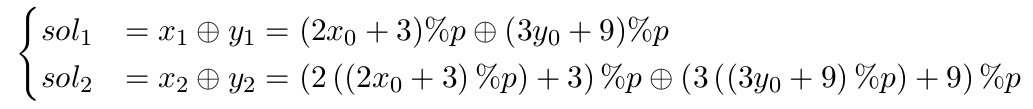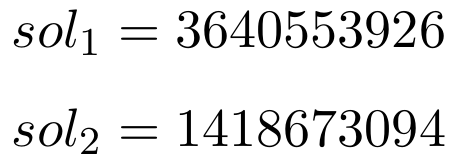I worked on this challenge with @dariosharp.

This crypto challenge (https://giant-goannas.ctfcompetition.com/) has a quite easy requestSo we have to choose the right number n-times consecutively. If we make a mistake, we have to repeat this process from the beginning (obviously with different numbers). In the comment of the previous web page of the challenge we find this code

``````class SecurePrng(object):
def __init__(self):
# generate seed with 64 bits of entropy
self.p = 4646704883L
self.x = random.randint(0, self.p)
self.y = random.randint(0, self.p)

def next(self):
self.x = (2 * self.x + 3) % self.p
self.y = (3 * self.y + 9) % self.p
return (self.x ^ self.y)
``````

So let’s do some mathso assume that we know the first two correct number (just guess that): we only have to solve this system of equations in order to find the first two random integerWe guess the fist two step (not so difficult, it happens with 0.25 probability): in our case`Mathematica` refuses to solve that equation system: no problem, let’s use z3!

``````from z3 import *
import sys

s1cor = int(sys.argv)
s2cor = int(sys.argv)
dimVector =35
x = BitVec('x', dimVector)
y = BitVec('y', dimVector)
p = BitVec('p',dimVector)
s1 = BitVec('s1',dimVector)
s2 = BitVec('s2',dimVector)
s = Solver()
s.add( ( ( ( 2 * x + 3 ) % p ) ^ ( ( 3 * y + 9 ) % p ) )==s1)
s.add(( ( ( 2 * ( ( 2 * x + 3 ) % p )  + 3 ) % p ) ^ ( ( 3 * ( (  3 * y + 9 ) % p) + 9 ) % p ) )==s2)
while s.check() == sat:
class SecurePrng(object):
def __init__(self,x,y):
self.p = 4646704883L
self.x = x
self.y = y
def next(self):
self.x = (2 * self.x + 3) % self.p
self.y = (3 * self.y + 9) % self.p
return (self.x ^ self.y)
def getX(self):
return self.x
def getY(self):
return self.y
m = s.model()
pMy = 4646704883L
myObj = SecurePrng(int(str(m[x]))%pMy,int(str(m[y]))%pMy)
mySol1 = myObj.next()
mySol2 = myObj.next()
if mySol1 == s1cor and mySol2 == s2cor and int(str(m[x]))<= pMy and int(str(m[y])) <= pMy :
print "x = " + str(m[x]) + " ; y = " + str(m[y])
s.add(Or(x != s.model()[x], y != s.model()[y]))
``````

just few notes: there are more than one solution of that system, so we check every solution that z3 proposes.

Run that s**t!

``````\$ time python solver.py 3640553926 1418673094
x = 3714993585 ; y = 2248563082

real	0m0.591s
user	0m0.556s
sys	0m0.012s
``````

ok: we found the two random seed and and we were able to generate all the solution.

``````>>> import random
>>>
>>> class SecurePrng(object):
...     def __init__(self):
...         self.i = 0
...         self.p = 4646704883L
...         self.x = 3714993585 % self.p
...         self.y = 2248563082 % self.p
...     def next(self):
...         print self.i
...         self.i += 1
...         self.x = (2 * self.x + 3) % self.p
...         self.y = (3 * self.y + 9) % self.p
...         return (self.x ^ self.y)
...     def getX(self):
...             return self.x
...     def getY(self):
...             return self.y
...
>>>
>>> mySol = SecurePrng()
>>> print "x0 = " + str(mySol.getX())
x0 = 3714993585
>>> print "y0 = " + str(mySol.getY())
y0 = 2248563082
>>> print mySol.next()
0
3640553926
>>> print "x1 = " + str(mySol.getX())
x1 = 2783282290
>>> print "y1 = " + str(mySol.getY())
y1 = 2098984372
>>> print mySol.next()
1
1418673094
>>> print mySol.next()
2
2142807111
>>> print mySol.next()
3
3977384148
...
...
>>> print mySol.next()
100
31332507
``````

After 100 step we get the flag CTF{!_aRe_y0U_tH3_NSA:-?!}

Solver for this challenge can be found here.

Written on April 30, 2016